# Working out the current and voltage in a circuit

Bolter
Homework Statement:
Calculate the circuit's current and pd across a component
Relevant Equations:
Ohm's law
Here are a few problems I have been trying which are shown below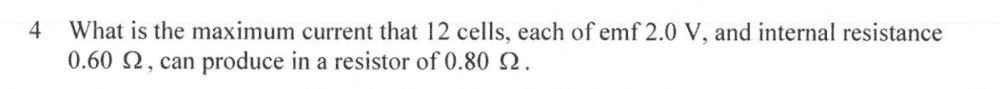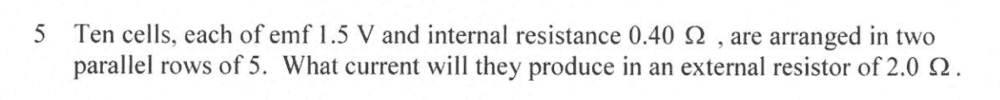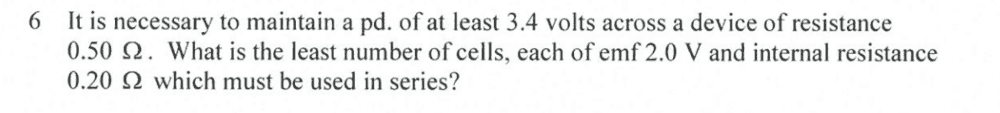Here is what I have done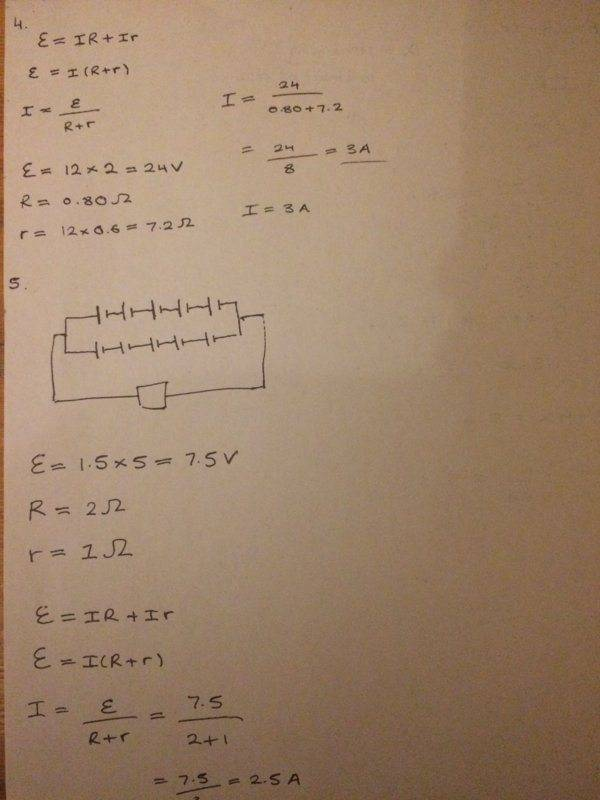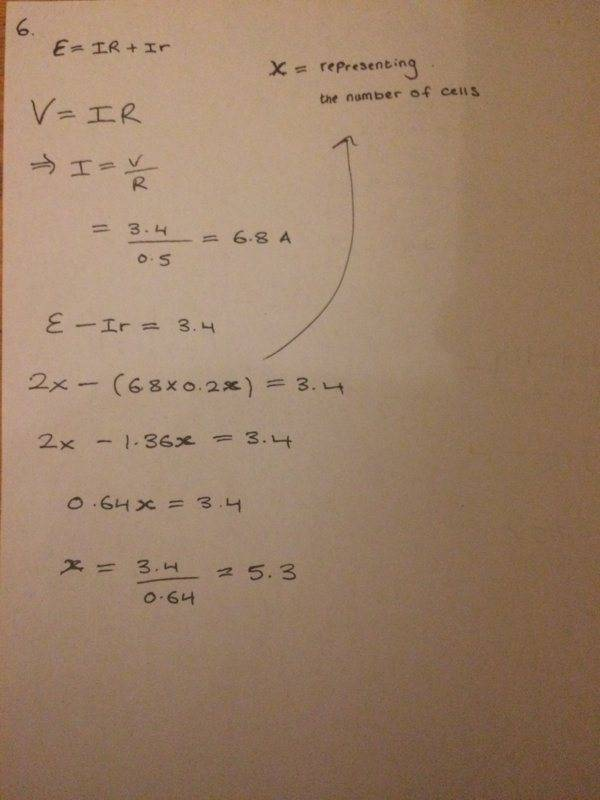The correct answers for these Q's are: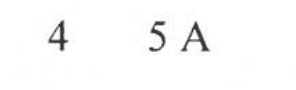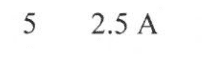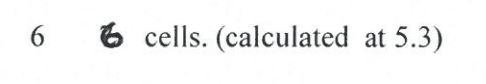I'm not sure what I have done wrong for Q4, I assumed that all 12 cells were connected in series thus each individual emf sums up to 24V
Similarly the internal resistances must sum up to 7.2 ohms

Is there anything that I am missing for me to get a current value of 5 amps instead of 3 amps?

Any help would be really appreciated! Thanks

Homework Helper
Gold Member
2022 Award
I'm not sure what I have done wrong for Q4, I assumed that all 12 cells were connected in series
Bear in mind that if the cells are in series then so are their internal resistances.

•DaveE
Gold Member
Your calculation is correct, given the assumptions you made about the configuration of the circuit elements. Try some other arrangements to see if you've found the best one.

Bolter
Your calculation is correct, given the assumptions you made about the configuration of the circuit elements. Try some other arrangements to see if you've found the best one.

I believe that the maximum current can only be drawn out when all cells are attached in series, as this is the only way to produce the biggest EMF.
Arranging some or all cells in parallel would give a smaller EMF off

So biggest current has to be 3 amps then?

Homework Helper
Similarly the internal resistances must sum up to 7.2 ohms
Hint: this also means that all current has to pass through all internal resistances -- not so favourable.

Homework Helper
So biggest current has to be 3 amps then?
NopeBolter
Hint: this also means that all current has to pass through all internal resistances -- not so favourable.

Would this be a case of trial and error in figuring out what configuration the circuit elements must be to give a current of 5A?

So there has to be some parallel arrangement involved I suppose to decrease the total internal resistance since I=V/R, if R gets smaller then surely a bigger current is drawn. However that would also mean the emf, V gets smaller too?

Homework Helper
as this is the only way to produce the biggest EMF.
biggest EMF at zero current - you found that 90% of that EMF is lost on internal resistances !

Homework Helper
Would this be a case of trial and error in figuring out what configuration the circuit elements must be to give a current of 5A?
Basically: yes. I can't think of a 'golden rule'. In electronics there is somethinig called 'impedance matching', but for this exercise it doesn't work ---  or does it ?

I, for example started with a try of all 12 parallel -- then the internal power loss is minimal, but the final EMF is too low. Fortunately there are only five sensible variations.

Bolter
Basically: yes. I can't think of a 'golden rule'. In electronics there is somethinig called 'impedance matching', but for this exercise it doesn't work ---  or does it ?

I, for example started with a try of all 12 parallel -- then the internal power loss is minimal, but the final EMF is too low. Fortunately there are only five sensible variations.

I tried one other variation now where the cells are arranged in two parallel rows of 6, and this set up is then in series with the 0.8 ohm resistor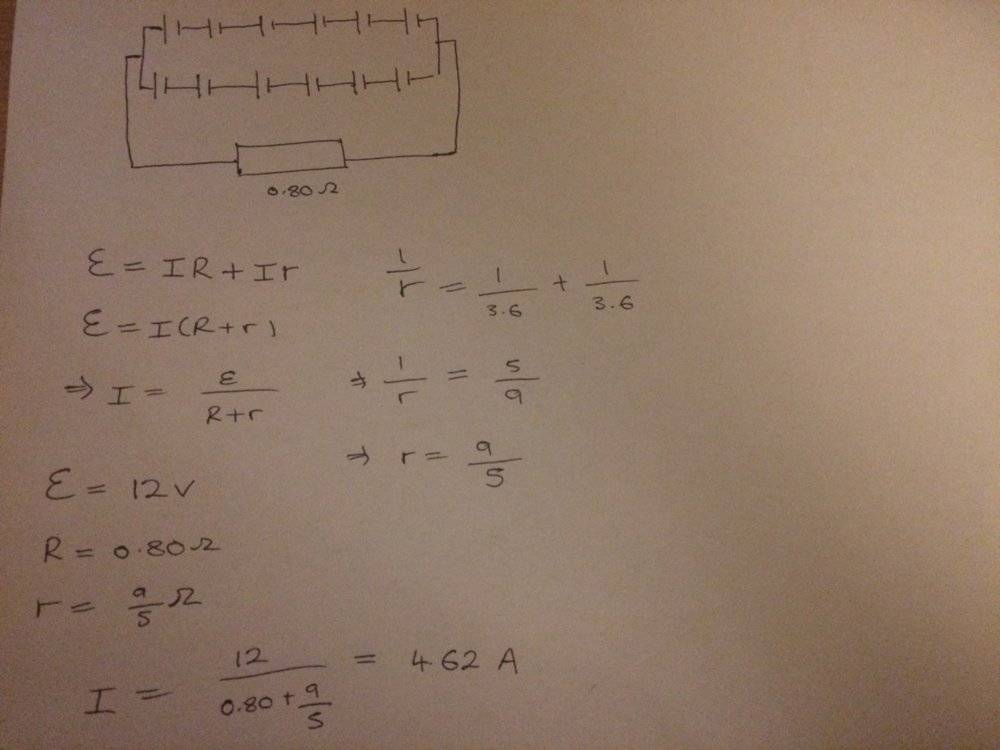I get a new current of 4.62 A which is close to 5 A but not exactly 5 AHomework Helper
Keep going ...
You tried 12 x 1 with 24 V, 7.2 ##\Omega## internal resistance and 6 x 2 with 12 V, 1.8 ##\Omega## internal resistance.
I tried 1 x 12 with 2 V, 0.05 ##\Omega## internal resistance. Three down, two to go ...

Bolter
Keep going ...
You tried 12 x 1 with 24 V, 7.2 ##\Omega## internal resistance and 6 x 2 with 12 V, 1.8 ##\Omega## internal resistance.
I tried 1 x 12 with 2 V, 0.05 ##\Omega## internal resistance. Three down, two to go ...

Is this some sort of pattern that I'm not seeing?

I tried with 2 more possible set ups
Where one involved 4 parallel rows of 3 cells in series with fixed resistor
and another involved 3 parallel rows of 4 cells in series with fixed resistor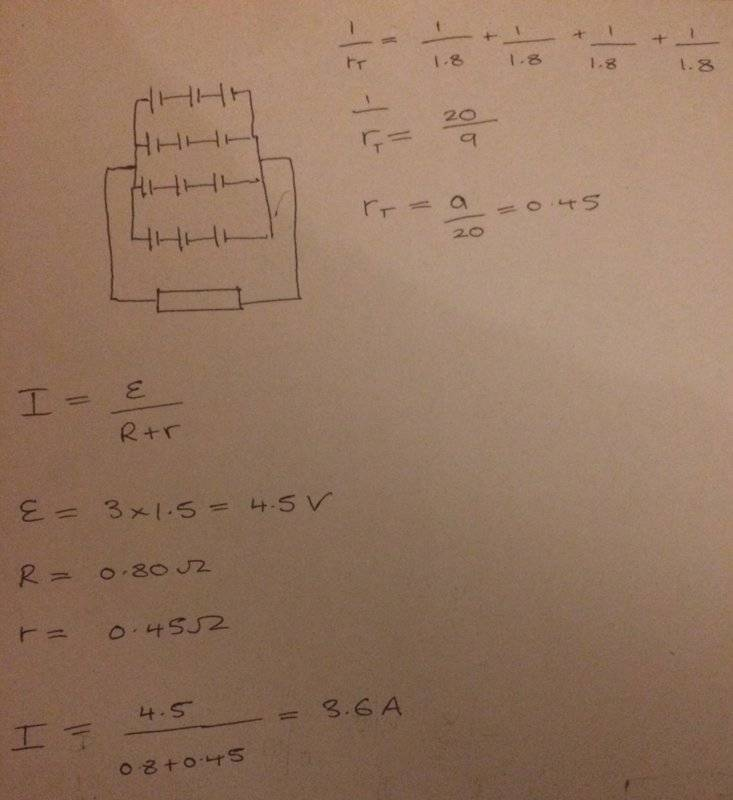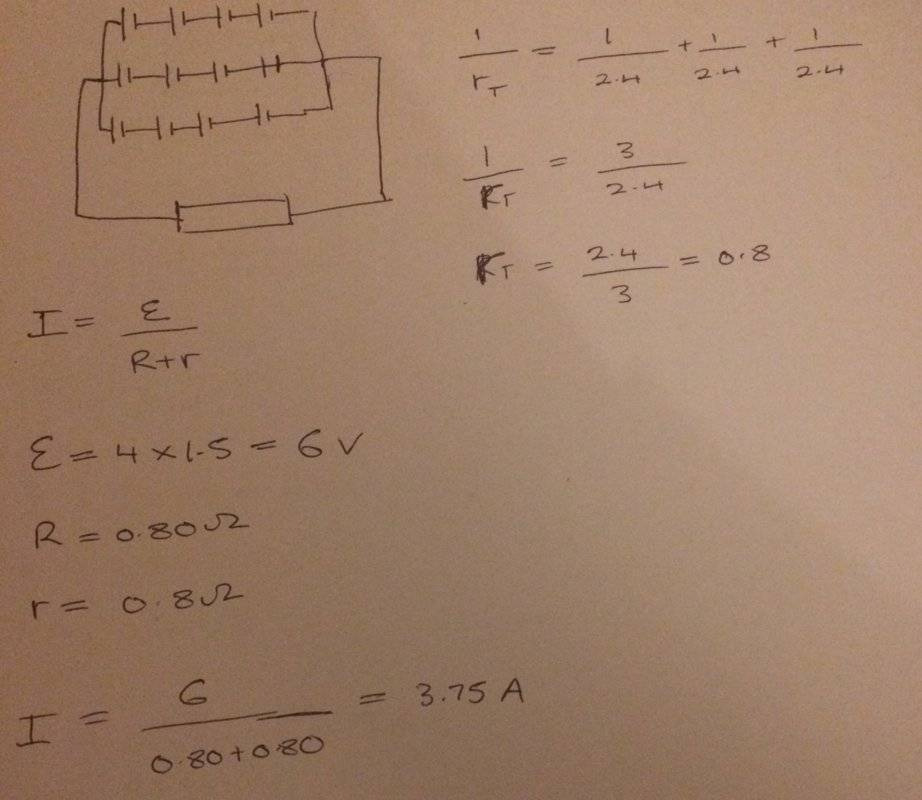But the currents that I obtain from both are either 3.6 A or 3.75 A (not close to 5 A)

Homework Helper
2022 Award
These are designated as 2V cells. (!)

•Bolter
Homework Helper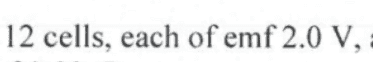get some rest !

•Bolter and hutchphd
Homework Helper
Gold Member
2022 Award
Would this be a case of trial and error
Not entirely.
In posts #10 and #12 you tried rectangular arrangements. You can generalise this to m by n, where mxn=12. It is not hard to figure out the current as a function of m.

Bolter
View attachment 256008

get some rest !

Oh shoot yes you're right!
My brain hasn't been processing properly which is why I mistook the value, actually was feeling tiredI tried with the emf of cell being 2.0 V now and get current to be 4.8 A for the circuit with 4 parallel rows of 3 cells
and 5 A with 3 parallel rows of 4 cells.

So the last configuration must be the one I am looking for to use, to generate a circuit current of 5 A

Bolter
Not entirely.
In posts #10 and #12 you tried rectangular arrangements. You can generalise this to m by n, where mxn=12. It is not hard to figure out the current as a function of m.

Right I now see, so all possible combinations I could have used are:

12 cells all in series
2 parallel rows of 6 cells
4 parallel rows of 3 cells
3 parallel rows of 4 cells
6 parallel rows of 2 cells

And then finally all 12 cells in parallel with each other so we have 12 rows then

so for mxn=12

I know m denotes the number of branches
and n denotes the number of cells in that branch

What do you mean when you say current can written as a function of m

I know that in parallel arrangements, current is known to split when it comes to an entry junction however same amount of current must leave at exit junction too. This is just Kirchhoff's 1st law that I am stating.

Looking at my case, I believe I will have equal amount of current flowing through all branches. This is since I'm dealing with identical cells all which has same number of cells on each branch. Current has to shared proportionately from resistance available in each row, right?

Is this the sort of information I have to use to formulate a function of m to give current?

Last edited:
Homework Helper
And it so happens that in the 4 x 3 case the internal impedance is 0.8 ##\Omega## ...
Must be more than just a coincidence ...

•hutchphd
Bolter
And it so happens that in the 4 x 3 case the internal impedance is 0.8 ##\Omega## ...
Must be more than just a coincidence ...

Yep, should really look into this. Would come into be nifty at times

Homework Helper
Gold Member
2022 Award
What do you mean when you say current can written as a function of m
Let the current in each branch be I and end to end voltage be V.
If the external resistance is R, what equation relates V, I, m and R?
If the internal resistance is r and the internal voltage is v, what equation relates V, v, n, I and r?

•BvU
Bolter
Let the current in each branch be I and end to end voltage be V.
If the external resistance is R, what equation relates V, I, m and R?
If the internal resistance is r and the internal voltage is v, what equation relates V, v, n, I and r?

Looking at the whole circuit, isn't external resistance R just R = V/I. I can't see how m gets related

And focusing on one single branch, where let's say there are 'n' number of cells in that branch

v = nIr
so V + v = IR + nIr = I (R + nr)

Edit: Is V = mIR?

As a same current I, is flowing through 'm' number of branches, all these currents then get combined at the the end of a node to produce a current of mI. Thus R = V/mI

Last edited:
Homework Helper
Gold Member
2022 Award
v = nIr
No. In each cell in the line you have an emf of v partly used up by the emf needed to push a current I through internal resistance r.
But it isn't all used up or V would be 0.
Is V = mIR?
Yes.

Bolter
No. In each cell in the line you have an emf of v partly used up by the emf needed to push a current I through internal resistance r.
But it isn't all used up or V would be 0.

Yes.

Hmm ok so terminal voltage (V) + lost volts (v) = emf

Then v = emf – V

v = emf – mIR

I would express emf as 2n, where 2 is the emf of a single cell and 'n' is the number of cells in that line

Therefore v = 2n – mIR?

Homework Helper
Gold Member
2022 Award
Hmm ok so terminal voltage (V) + lost volts (v) = emf

Then v = emf – V

v = emf – mIR

I would express emf as 2n, where 2 is the emf of a single cell and 'n' is the number of cells in that line

Therefore v = 2n – mIR?
No, I defined v as the nominal voltage (2 Volts) of each cell. Of that, Ir is lost in each cell leaving ...? Adding that up for the line of n cells gives ...? Which is equal to what other variable?

•Bolter
Bolter
No, I defined v as the nominal voltage (2 Volts) of each cell. Of that, Ir is lost in each cell leaving ...? Adding that up for the line of n cells gives ...? Which is equal to what other variable?

So it should be nv – nIr = V

factoring out n gives n(v – Ir) = V

Homework Helper
Gold Member
2022 Award
So it should be nv – nIr = V

factoring out n gives n(v – Ir) = V
Right.
Combine that with your other equation for V to get mI (the total current) in terms of n, m, v, r and R.

Bolter
Right.
Combine that with your other equation for V to get mI (the total current) in terms of n, m, v, r and R.

Combining it, I get this,
where I do get 5A once I let m = 3 and n = 4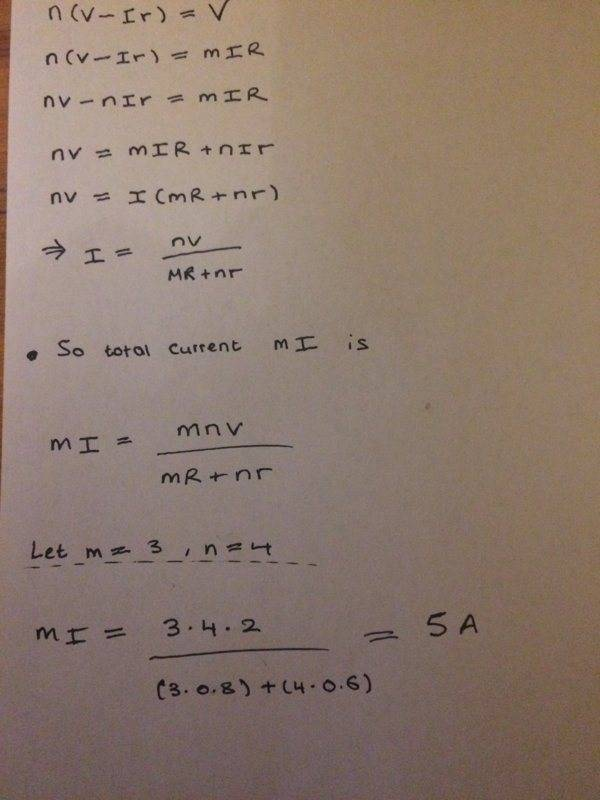Homework Helper
Gold Member
2022 Award
Combining it, I get this,
where I do get 5A once I let m = 3 and n = 4

View attachment 256077
Yes, but better still you can avoid having to guess. How do you find the maximum of a function?

•Bolter
Bolter
Yes, but better still you can avoid having to guess. How do you find the maximum of a function?

Work out the first order derivative of that function and equate it to zero. Though don't we have 2 unknowns in the function which are m and n? so you couldn't find either?

Homework Helper
Gold Member
2022 Award
Work out the first order derivative of that function and equate it to zero. Though don't we have 2 unknowns in the function which are m and n? so you couldn't find either?
The product mn is fixed, so there is only one independent variable.

Bolter
The product mn is fixed, so there is only one independent variable.

If product mn is fixed then it can treated as a constant which I'll label as k

Therefore I have total current = kv/mR + nr

What should I differentiate this with respect to?

Edit:

Would this be along the right lines? Where I differentiate with respect to n, set that derivative to zero to find n. Then subsequently get m by 12/n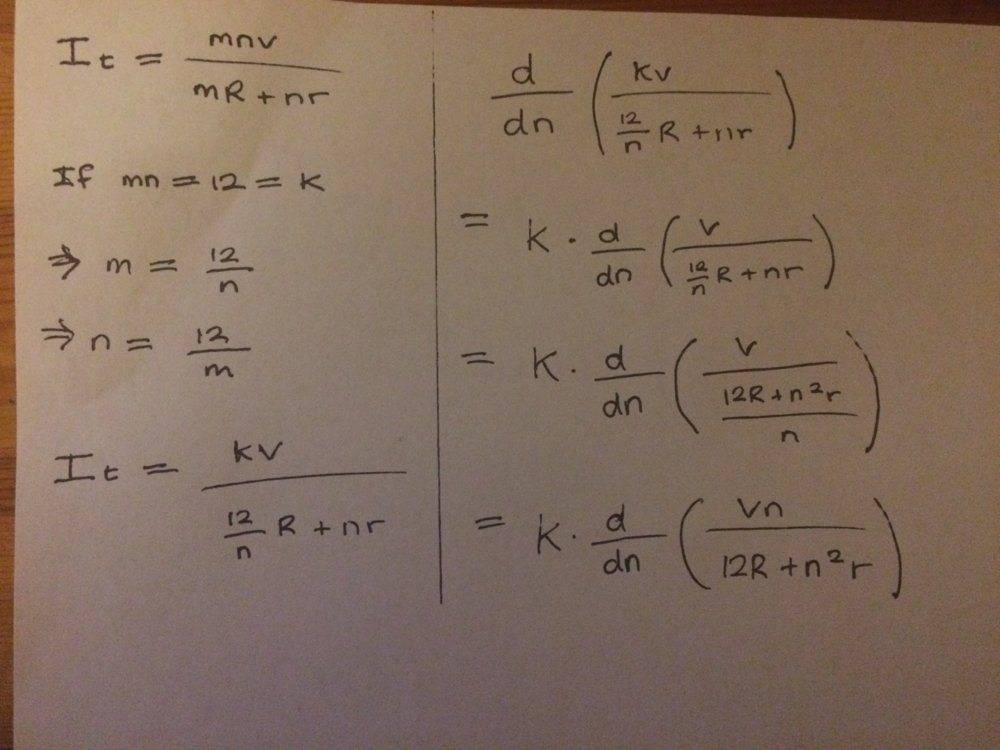Last edited:
Homework Helper
Gold Member
2022 Award
If product mn is fixed then it can treated as a constant which I'll label as k

Therefore I have total current = kv/mR + nr

What should I differentiate this with respect to?

Edit:

Would this be along the right lines? Where I differentiate with respect to n, set that derivative to zero to find n. Then subsequently get m by 12/n

View attachment 256081
Yes, that looks right. But why use K in some places and 12 in others?

Bolter
Yes, that looks right. But why use K in some places and 12 in others?

Yes sorry, should've stayed consistent and used k or 12 only throughout.

To differentiate the function I used quotient rule to do this and solve for n and m when letting the derivative be set to 0

And I do get n = 4 and m = 3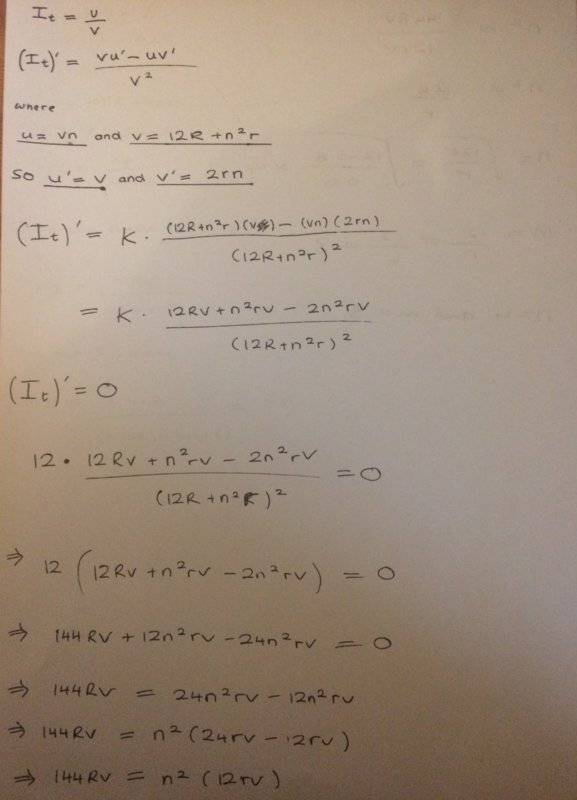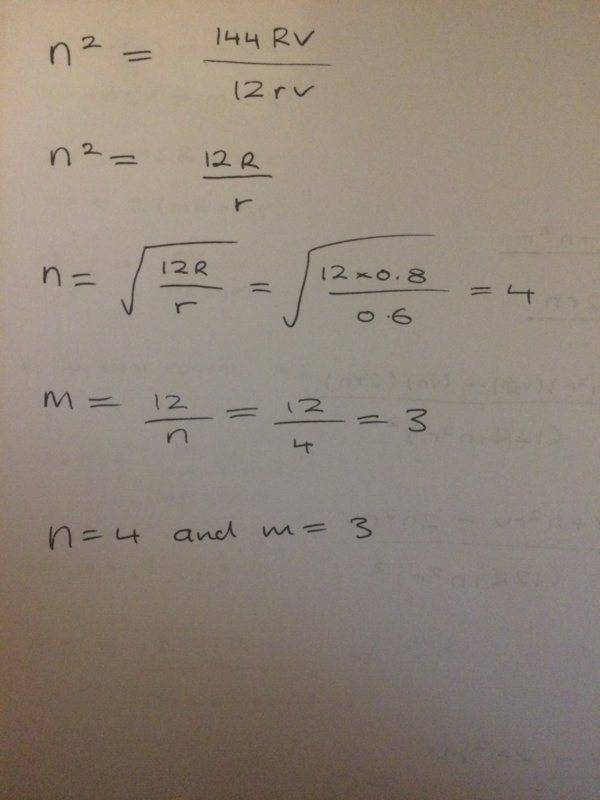Homework Helper
Gold Member
2022 Award
Yes sorry, should've stayed consistent and used k or 12 only throughout.

To differentiate the function I used quotient rule to do this and solve for n and m when letting the derivative be set to 0

And I do get n = 4 and m = 3

View attachment 256082
View attachment 256083
Very good.
Note that the m:n ratio turns out always to be the ratio of the resistances, with the higher internal resistance corresponding to the wider, shorter array.

•BvU and Bolter